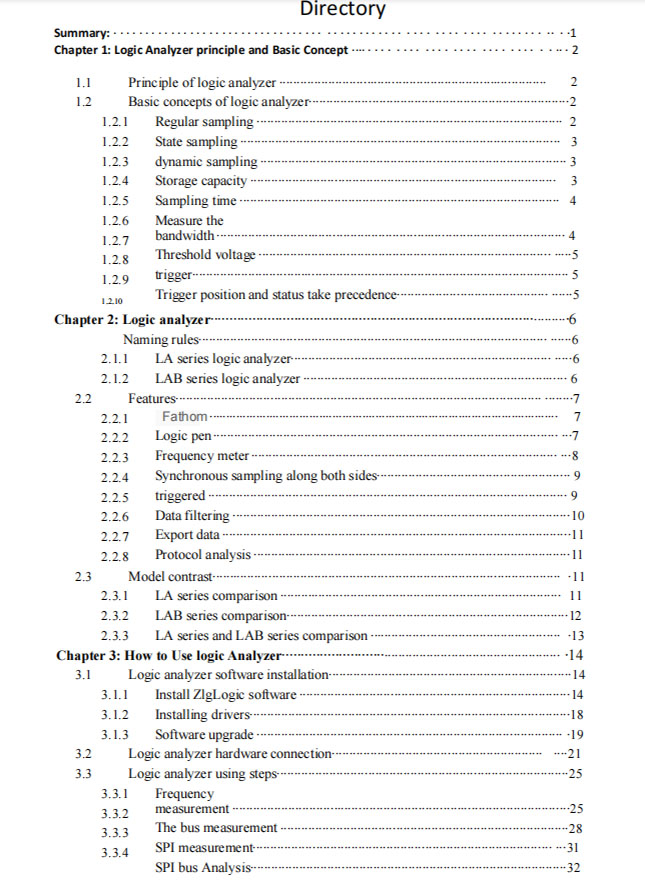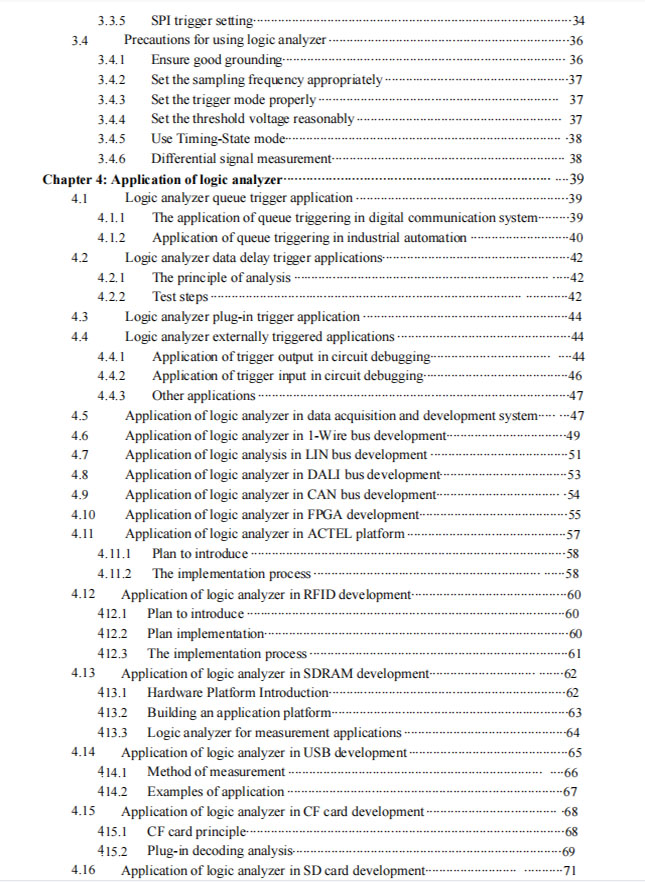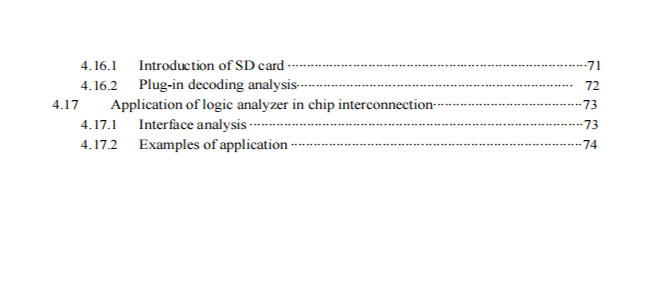# Logical analysis still Uses Instructions for USB flash drive SD TF card data recovery tools

\$99.00

An overview of the

With the rapid development of electronic technology, the proportion of digital circuit in electronic

development is increasing, how to effectively search and verify the digital circuit error is becoming

more and more important. Logic analyzer, as a tool to analyze the logic level of digital circuit, is

mainly used to locate the specific waveform data of system operation error, and infer the cause of the

system error by observing the waveform data, so as to find out the solution to the error.

Logic analyzer can be divided into four levels in application:

(1) Observe the waveform: whether there are burrs and interference in the waveform, and whether the frequency is correct;

(2) Timing sequence measurement: timing sequence analysis of the measured signals to eliminate operational conflicts,

timing sequence coordination and other problems;

(3) Auxiliary analysis: analyze bus signals or advanced protocols with the complete analysis function of logic analyzer;

(4) Error elimination: the use of logic analyzer powerful trigger function to capture errors, exclude hidden errors in the

system.

This paper is mainly explained from the following aspects:

(1) Chapter I: Logic analyzer principle and basic concept;

(2) Chapter two: Zhiyuan logic analyzer;

(3) Chapter 3: How to use logic analyzer;

(4) Chapter 4: Application of logic analyzer;

(5) Chapter 5: Logic analyzer case sharing.

In these chapters, we need to focus on the first three chapters, through learning to understand the

basic concept of the logic analyzer, understand the functional characteristics of zhiyuan logic

analyzer and the difference between each model, know how to use the logic analyzer, so as to achieve

the correct selection for customers, explain and demonstrate the effect. The fourth chapter through

some applications to help you find and dig potential customers in various research and development

fields, the fifth chapter can facilitate the exchange of experience in the sales of logic analyzer, we

need to provide materials for sorting, these two chapters we only need to understand. Finally, I wish

you all a happy study!## Reviews

There are no reviews yet.

Shopping Cart